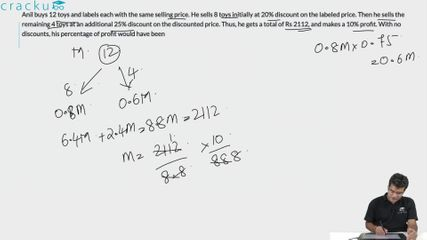Question 66

# Anil buys 12 toys and labels each with the same selling price. He sells 8 toys initially at 20% discount on the labeled price. Then he sells the remaining 4 toys at an additional 25% discount on the discounted price. Thus, he gets a total of Rs 2112, and makes a 10% profit. With no discounts, his percentage of profit would have been

Solution

Let the CP of the each toy be "x". CP of 12 toys will be "12x". Now the shopkeeper made a 10% profit on CP. This means that

12x(1.1)= 2112 or x=160 . Hence the CP of each toy is ₹160.

Now let the SP of each toy be "m". Now he sold 8 toys at 20% discount. This means that 8m(0.8) or 6.4m

He sold 4 toys at an additional 25% discount. 4m(0.8)(0.75)=2.4m  Now 6.4m+2.4m=8.8m=2112 or m=240

Hence CP= 160 and SP=240. Hence profit percentage is 50%.

### View Video Solution